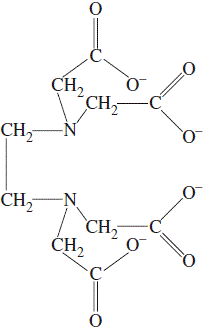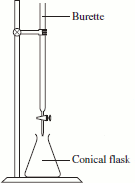Determining Calcium Ion Concentration in Water by Complexometric Titration Chemistry Tutorial

Key Concepts• Calcium and magnesium ions dissolved in water cause water hardness.
• Ethylenediaminetetraacetic acid (EDTA), shown on the right in its deprotonated form, is commonly used in a titration to determine the concentration of Ca2+ and Mg2+ ions in water because both ions form complexes with EDTA.
• In order to determine the concentration of Ca2+ in water, the Mg2+ must be removed first, usually by precipitation.
• The titration with EDTA then determines the concentration of Ca2+ remaining in the water.
• The complex formed between Ca2+ and EDTA is colourless and soluble, so a suitable indicator must be used to show the endpoint of the titration.
• The concentration of Ca2+ in water can then be calculated.

No ads = no money for us = no free stuff for you!

Precipitating the Magnesium Ions.

Calcium and magnesium belong to the same group in the Periodic Table, Group 2.
This means that the chemistry of calcium and magnesium is similar.
We need to choose a reagent to add to the water that forms an insoluble solid (a precipitate) with magnesium but not with calcium.
We can do this by comparing the solubility product, Ksp, values for a number of calcium and magnesium salts as shown in the table below:

Ksp
magnesiumcalcium
carbonate3.5 x 10-82.8 x 10-9
oxalate1.0 x 10-84.0 x 10-9
fluoride6.5 x 10-94.0 x 10-11
hydroxide1.8 x 10-115.0 x 10-6

Magnesium carbonate, oxalate and fluoride are all more soluble than calcium carbonate, oxalate and fluoride.

Magnesium hydroxide, however, is less soluble than calcium hydroxide.

It should be possible to precipitate magnesium hydroxide out of the water without precipitating out all the calcium as calcium hydroxide.

 Mg(OH)2Mg2+(aq) + 2OH-(aq) Ksp(Mg(OH)2) =[Mg2+][OH-]2 1.8 x 10-11 = [Mg2+][OH-]2 Let y = [Mg2+] at equilibrium Then [OH-] = 2y at equilibrium So 1.8 x 10-11 = y x (2y)2 1.8 x 10-11 = y x 4y2 1.8 x 10-11 = 4y3 4.5 x 10-12 = y3 y = 1.65 x 10-4 [Mg2+] = 1.65 x 10-4 mol L-1 [OH-] = 2 x 1.65 x 10-4 = 3.30 x 10-4 mol L-1 Ca(OH)2Ca2+(aq) + 2OH-(aq) Ksp(Ca(OH)2) =[Ca2+][OH-]2 5.0 x 10-6 = [Ca2+][OH-]2 Let y = [Ca2+] at equilibrium Then [OH-] = 2y at equilibrium So 5.0 x 10-6 = y x (2y)2 5.0 x 10-6 = y x 4y2 5.0 x 10-6 = 4y3 1.25 x 10-6 = y3 y = 1.08 x 10-2 [Ca2+] = 1.08 x 10-2 mol L-1 [OH-] = 2 x 1.08 x 10-2 = 2.16 x 10-2 mol L-1 Under the same conditions, the concentration of hydroxide ions in the calcium hydroxide solution is about 100 times more than the concentration of hydroxide ions in the magnesium hydroxide solution. Calcium hydroxide is more soluble than magnesium hydroxide under the same conditions.

An aqueous solution of sodium hydroxide can be used to precipitate out the magnesium hydroxide.

 Assume that hard water contains a maximum 1 g L-1 Mg2+ and 1 g L-1 Ca2+ To precipitate out Mg(OH)2, its ion product, Q, must be greater than its solubility product, Ksp. [Mg2+] = moles ÷ volume (L) [Mg2+] = 1/24.31 ÷ 1 = 0.041 mol L-1 Q = [Mg2+][OH-]2 > Ksp [Mg2+][OH-]2 > 1.8 x 10-11 [0.041][OH-]2 > 1.8 x 10-11 [OH-]2 > 4.4 x 10-10 mol L-1 [OH-] > 2.1 x 10-5 mol L-1 To precipitate out Ca(OH)2, its ion product, Q, must be greater than its solubility product, Ksp. [Ca2+] = moles ÷ volume (L) [Ca2+] = 1/40.08 ÷ 1 = 0.025 mol L-1 Q = [Ca2+][OH-]2 > Ksp [Ca2+][OH-]2 > 5.0 x 10-6 [0.025][OH-]2 > 5.0 x 10-6 [OH-]2 > 2.0 x 10-4 mol L-1 [OH-] > 1.4 x 10-2 mol L-1 In order to precipitate out Mg(OH)2 the [OH-] must be greater than 2.1 x 10-5 mol L-1, but [OH-] must be less than 1.4 x 10-2 so that the Ca(OH)2 does NOT precipitate out. The pH of the water sample should be adjusted to be about 12, at 25oC, using NaOH(aq) to precipitate out Mg(OH)2 without precipitating out Ca(OH)2.

Do you know this?

Play the game now!

The Titration

After the magnesium ions have been precipitated out of the hard water by the addition of NaOH(aq) to form white Mg(OH)2(s), the remaining Ca2+ ions in solution are titrated with EDTA solution.• The burettte is filled with an EDTA solution of known concentration.
Concentrations of 0.010 mol L-1 are commonly used.
• A suitable aliquot of hard water is placed in the conical flask.
For example, a 50.0 mL transfer pipette can be used to put the water sample into the flask.
• A drop of a suitable indicator is added to the water sample.
For example, Patton and Reeder's Indicator (also abbreviated to HHSNNA)
Patton and Reeder's Indicator will be red in the hard water sample and blue when excess EDTA has been added.
• EDTA is added from the burette to the conical flask until the indicator changes colour, indicating that the endpoint has been reached.
• The volume of EDTA used is recorded.
• The steps above are repeated until three concordant titres are obtained, that is, three volumes of EDTA that agree to within a drop, or 0.05 mL, of each other.
• The average of these concordant titres is then used to calculate the concentration of calcium ions in the hard water.

The Calculations

The balanced chemical equation for the reaction between Ca2+ and EDTA is shown below:
Ca2+ + H2EDTA2- → CaEDTA2- + 2H+

The stoichiometric (mole) ratio of Ca2+ : EDTA is 1 : 1

That is, at the equivalence point, the moles of EDTA added to the water equals the moles of Ca2+ originally present in the water
At the equivalence point: moles Ca2+ = moles EDTA

moles of EDTA = EDTA concentration (mol L-1) x volume EDTA used (L)

volume used = average of the concordant titres (L)

moles Ca2+ in hard water sample = moles EDTA used = EDTA concentration (mol L-1) x volume EDTA used (L)

concentration (molarity) of Ca2+ in hard water = moles Ca2+ ÷ volume of water sample used (L)

[Ca2+] = (EDTA concentration (mol L-1) x volume EDTA used (L)) ÷ volume water sample (L)

Do you understand this?

Take the test now!

Worked Example

A student has been asked to determine the concentration of calcium ions, in mg L-1, in a 1 L sample of water.
The student places 50.0 mL of the water sample in a conical flask.
NaOH(aq) is added to the sample until the pH is about 12. The solution is swirled for a couple of minutes to completely precipitate all the magnesium ions as white Mg(OH)2(s).
A drop of Patton and Reeder's Indicator is added to the water sample. The water sample changes colour to red.
The burette is filled with 50.00 mL of 0.010 mol L-1 EDTA solution.
The student conducts a rough titration and discovers the endpoint of the titration, when the indicator changes colour to blue, occurs when about 9 mL of EDTA have been added to the water.
The student then conducts 3 more titrations, taking great care.
The results of these titrations are shown below.

Titration no.volume of EDTA (mL)
18.76 mL
28.80 mL
38.78 mL

Calculate the concentration of calcium ions in the hard water sample.

• Calculate the average titre of EDTA:
average titre = (8.76 + 8.80 + 8.78) ÷ 3 = 8.78 mL = 8.78 x 10-3 L
• Calculate the moles of EDTA in the average titre:
moles(EDTA) = concentration (mol L-1) x volume (L) = 0.010 x 8.78 x 10-3 = 8.78 x 10-5 mol
• Calculate moles of Ca2+ present in the 50.0 mL water sample:
Ca2+ + H2EDTA2- → CaEDTA2- + 2H+
moles Ca2+ = moles EDTA = 8.78 x 10-5 mol
• Calculate concentration (molarity) of calcium ions in the water:
molarity(Ca2+) = moles ÷ volume (L) = 8.78 x 10-5 ÷ 50.0 x 10-3 = 1.76 x 10-3 mol L-1
• Convert the concentration in mol L-1 to a concentration in mg L-1:
1 L of water contains 1.76 x 10-3 moles Ca2+
mass Ca2+ = moles x molar mass = 1.76 x 10-3 x 40.08 = 7.05 x 10-2 g
convert mass in grams to mass in mg by multiplying by 1000:
7.05 x 10-2 g = 7.05 x 10-2 x 1000 mg/g = 70.5 mg
concentration of Ca2+ = 70.5 mg L-1

Can you apply this?

Join AUS-e-TUTE!

Do the drill now!